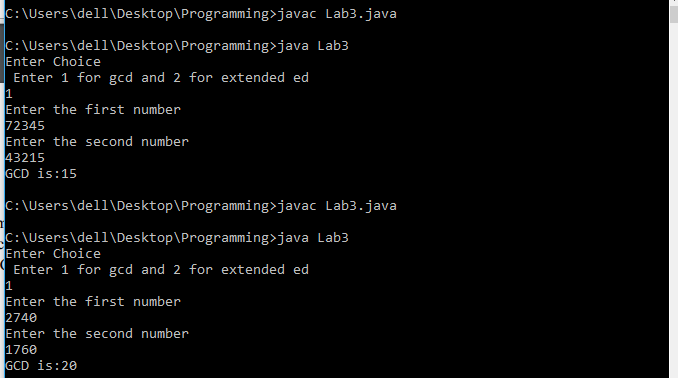# Code Examples

## Implement the Pseudo-codes of Euclid’s AlgorithmLet us look into how to implement Euclid’s algorithm and Euclid’s Extended Algorithms using Java Programming Language. Euclid’s Algorithm Pseudocode Euclid’s Extended Algorithm Pseudocode Question Implement the pseudo-codes of Euclid’s algorithm with recursive function and extended Euclid’s algorithm in any programming language you are comfortable with. Your program should take two integers A and B …

## C Program to Find GCD

C Program to Find GCD: This post discusses how to write C program to find GCD. There are many approaches to find the greatest common divisor (GCD) of any two numbers. It is the highest number that can divide both the numbers. The commonly used method to find the GCD of any two numbers is …

## C Program to Print Fibonacci Numbers

Before looking into the program to print Fibonacci numbers, let us look into what really it is.  Fibonacci numbers are a sequence of numbers known as a Fibonacci sequence which is characterized by the fact that ‘every number after the first two is the sum of the two preceding ones’ as shown below: 1, 1, 2, 3, 5, 8, 13, …